# NCERT Solutions for Class 7 Maths Chapter 13 Exponents and Powers (EX 13.1) Exercise 13.1

## NCERT Solutions for Class 7 Maths Chapter 13 Exponents and Powers (EX 13.1) Exercise 13.1

Free PDF download of NCERT Solutions for Class 7 Maths Chapter 13 Exercise 13.1 (EX 13.1) and all chapter exercises at one place prepared by expert teacher as per NCERT (CBSE) books guidelines. Class 7 Maths Chapter 13 Exponents and Powers Exercise 13.1 Questions with Solutions to help you to revise complete Syllabus and Score More marks. Register and get all exercise solutions in your emails. Every NCERT Solution is provided to make the study simple and interesting on Vedantu. Subjects like Science, Maths, English will become easy to study if you have access to NCERT Solution for Class 7 Science , Maths solutions and solutions of other subjects.

Do you need help with your Homework? Are you preparing for Exams?
Study without Internet (Offline)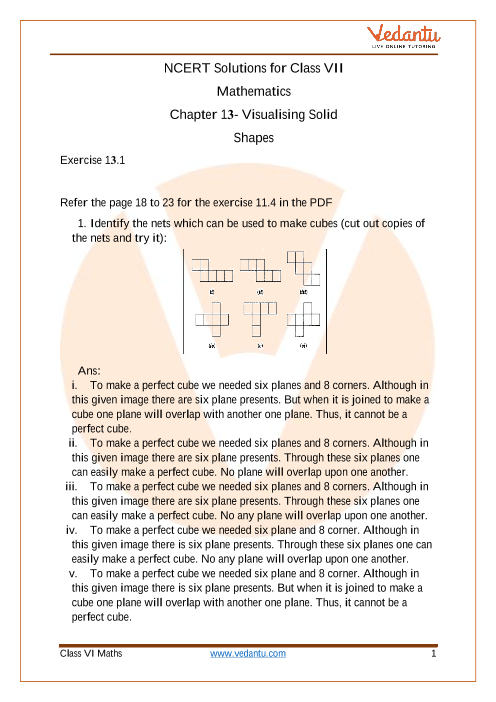Book your Free Demo session
Get a flavour of LIVE classes here at Vedantu## Access NCERT Solution for Class 7 Chapter 13- Exponents and powers

Exercise 13.1

Refer to exercise 13.1 in the PDF

1. Find the value of:

i) ${2^6}$

Ans: The given expression is : ${2^6}$

By expanding the term ${2^6}$ we get,

${2^6} = 2 \times 2 \times 2 \times 2 \times 2 \times 2$

By multiplying,

${2^6} = 64$

Hence, the required value of ${2^6}$ is $64$.

ii) ${9^3}$

Ans: The given expression is : ${9^3}$

By expanding the term ${9^3}$ we get,

${9^3} = 9 \times 9 \times 9$

By multiplying,

${9^3} = 729$

Hence, the required value of ${9^3}$ is $729$.

iii) ${11^2}$

Ans: The given expression is : ${11^2}$

By expanding the term ${11^2}$ we get,

${11^2} = 11 \times 11$

By multiplying,

${11^2} = 121$

Hence, the required value of ${11^2}$ is $121$.

iv) ${5^4}$

Ans: The given expression is : ${5^4}$

By expanding the term ${5^4}$ we get,

${5^4} = 5 \times 5 \times 5 \times 5$

By multiplying,

${5^4} = 625$

Hence, the required value of ${5^4}$ is $625$.

2. Express the following in exponential form:

i) $6 \times 6 \times 6 \times 6$

Ans: The given expression is : $6 \times 6 \times 6 \times 6$

The number of $6$’s in the given expression is $4$ .

So, the exponent of $6$ is $4$.

Hence, $6 \times 6 \times 6 \times 6 = {6^4}$.

ii) $t \times t$

Ans: The given expression is : $t \times t$

The number of $t$ ’s in the given expression is $2$.

So, the exponent of $t$ is $2$.

Hence, $t \times t = {t^2}$.

iii) $b \times b \times b \times b$

Ans: The given expression is : $b \times b \times b \times b$

The number of $b$ ’s in the given expression is $4$.

So, the exponent of $t$ is $4$.

Hence, $b \times b \times b \times b = {b^4}$.

iv) $5 \times 5 \times 7 \times 7 \times 7$

Ans: The given expression is : $5 \times 5 \times 7 \times 7 \times 7$

The number of $5$ ’s in the given expression is $2$ .

The number of $7$ ’s in the given expression is $3$.

So, the exponent of $5$ is $2$ and the exponent of $7$ is $3$.

Hence, $5 \times 5 \times 7 \times 7 \times 7 = {5^2} \times {7^3}$.

v) $2 \times 2 \times a \times a$

Ans: The given expression is : $2 \times 2 \times a \times a$

The number of $2$ ’s in the given expression is $2$ .

The number of $a$ ’s in the given expression is $2$.

So, the exponent of $2$ is $2$ and the exponent of $a$ is $2$.

Hence, $2 \times 2 \times a \times a = {2^2} \times {a^2}$.

vi) $a \times a \times a \times c \times c \times c \times c \times d$

Ans: The given expression is : $a \times a \times a \times c \times c \times c \times c \times d$

The number of $a$ ’s in the given expression is $3$.

The number of $c$ ’s in the given expression is $4$.

The number of $d$ ’s in the given expression is $1$.

So, the exponent of $a$ is $3$ , the exponent of $c$ is $4$ and the exponent of $d$ is $1$ .

Hence, $a \times a \times a \times c \times c \times c \times c \times d = {a^3} \times {c^4} \times {d^1}$.

3. Express each of the following numbers using the exponential notation:

i) $512$

Ans: By factorization method, we can find,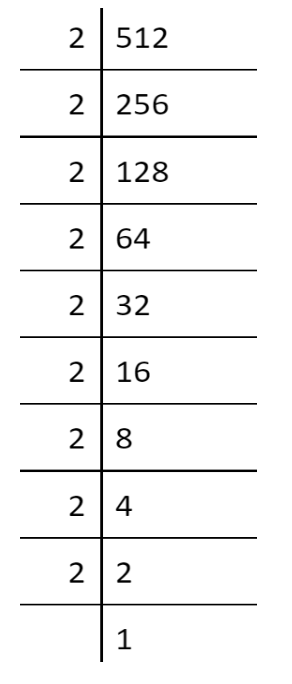In the above expanding form of $512$, numbers of $2$’s as a factor is $9$.

So, we can express $512$ as term of $2$ .

$512 = 2 \times 2 \times 2 \times 2 \times 2 \times 2 \times 2 \times 2 \times 2$

The exponent of $2$ will be $9$ .

Hence, $512 = {2^9}$.

ii) $343$

Ans: By factorization method we can find,

 7 343 7 49 7 7 1

In the above expanding form of $343$ , numbers of $7$’s as factor is $3$.

So, we can express $343$ as term of $7$ .

$343 = 7 \times 7 \times 7$

The exponent of $7$ will be $3$ .

Hence, $343 = {7^3}$.

iii) $729$

Ans: By factorization method we can find,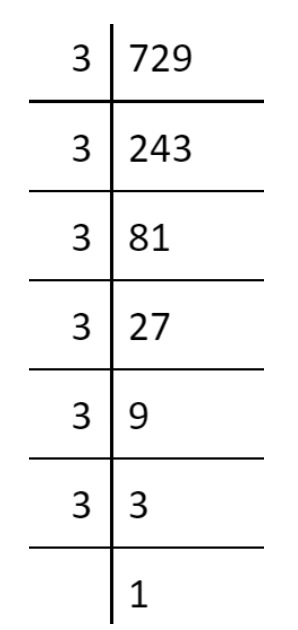In the above expanding form of $729$, numbers of $3$’s as the factor is $6$.

So, we can express $729$ as term of $3$ .

$729 = 3 \times 3 \times 3 \times 3 \times 3 \times 3$

The exponent of $3$ will be $6$ .

Hence, $729 = {3^6}$.

iv) $3125$

Ans: By factorization method, we can find,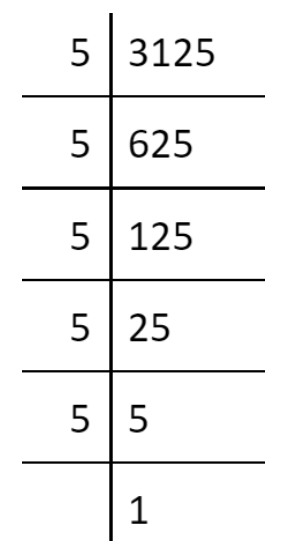In the above expanding form of $3125$, numbers of $5$’s as a factor is $5$.

So, we can express $3125$ as term of $5$ .

$3125 = 5 \times 5 \times 5 \times 5 \times 5$

The exponent of $5$ will be $5$ .

Hence, $3125 = {5^5}$.

4. Identify the greater number, wherever possible, in each of the following:

i) ${4^3}$ and ${3^4}$

Ans: ${4^3}$ can be written as,

${4^3} = 4 \times 4 \times 4$

${4^3} = 64$

${3^4}$can be written as,

${3^4} = 3 \times 3 \times 3 \times 3$

${3^4} = 81$

Since, $81 > 64$

Thus, ${3^4} > {4^3}$.

Hence, ${3^4}$ is greater than ${4^3}$.

ii) ${5^3}$ and ${3^5}$

Ans: ${5^3}$ can be written as,

${5^3} = 5 \times 5 \times 5$

${5^3} = 125$

${3^5}$ can be written as,

${3^5} = 3 \times 3 \times 3 \times 3 \times 3$

${3^5} = 243$

Since, $243 > 125$

Thus, ${3^5} > {5^3}$.

Hence, ${3^5}$ is greater than ${5^3}$.

iii) ${2^8}$ and ${8^2}$

Ans: ${2^8}$ can be written as,

${2^8} = 2 \times 2 \times 2 \times 2 \times 2 \times 2 \times 2 \times 2$

${2^8} = 256$

${8^2}$ can be written as,

${8^2} = 8 \times 8$

${8^2} = 64$

Since, $256 > 64$

Thus, ${2^8} > {8^2}$.

Hence, ${2^8}$ is greater than ${8^2}$.

iv) ${100^2}$ and ${2^{100}}$

Ans: ${100^2}$ can be written as,

${100^2} = 100 \times 100$

${100^2} = 10000$

${2^{100}}$can be written as,

${2^{100}} = 2 \times 2 \times 2 \times 2 \times 2 \times ....14{\text{ times}} \times .... \times 2$

${2^{100}} = 16384 \times ... \times 2$

Since, $16384 \times ... \times 2 > 10000$

Thus, ${2^{100}} > {100^2}$.

Hence, ${2^{100}}$ is greater than ${100^2}$.

v) ${2^{10}}$ and ${10^2}$

Ans: ${2^{10}}$can be written as,

${2^{10}} = 2 \times 2 \times 2 \times 2 \times 2 \times 2 \times 2 \times 2 \times 2 \times 2$

${2^{10}} = 1024$

${10^2}$ can be written as,

${10^2} = 10 \times 10$

${10^2} = 100$

Since, $1024 > 100$

Thus, ${2^{10}} > {10^2}$.

Hence, ${2^{10}}$ is greater than ${10^2}$.

5. Express each of the following as product of powers of their prime factors:

i) $648$

Ans: The given expression is $648$.

By factorization method we can find the prime factors,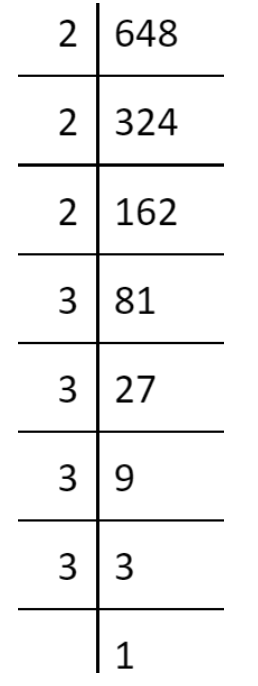So, $648$ can be written as

$648 = 2 \times 2 \times 2 \times 3 \times 3 \times 3 \times 3$

It can be express as exponent of $2$ and $3$ .

$648 = {2^3} \times {3^4}$

Hence, the required expression is $648 = {2^3} \times {3^4}$.

ii) $405$

Ans: The given expression is $405$.

By factorization method we can find the prime factors,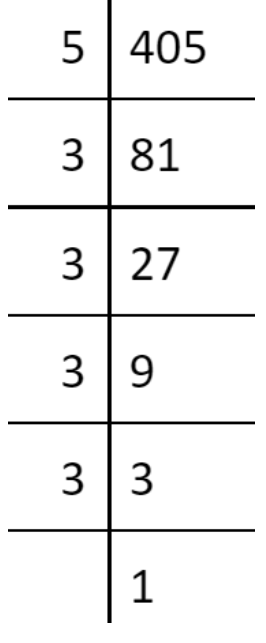So, $405$ can be written as

$405 = 5 \times 3 \times 3 \times 3 \times 3$

Now, it can be express as

$405 = 5 \times {3^4}$

Hence, the required expression is $405 = 5 \times {3^4}$.

iii) $540$

Ans: The given expression is $540$.

By factorization method we can find the prime factors,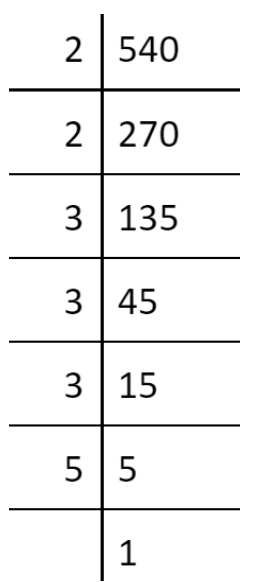So, $540$ can be written as

$540 = 2 \times 2 \times 3 \times 3 \times 3 \times 5$

Now, it can be express as

$540 = {2^2} \times {3^3} \times 5$

Hence, the required expression is $540 = {2^2} \times {3^3} \times 5$.

iv) $3600$

Ans: The given expression is $3600$.

By factorization method we can find the prime factors,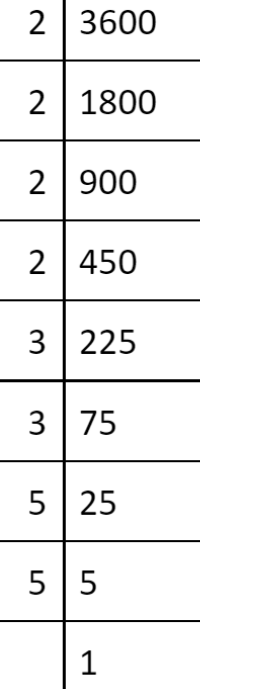2 3600 2 1800 2 900 2 450 3 225 3 75 5 25 5 5 1

So, $3600$ can be written as

$3600 = 2 \times 2 \times 2 \times 2 \times 3 \times 3 \times 5 \times 5$

Now, it can be express as

$3600 = {2^4} \times {3^2} \times {5^2}$

Hence, the required expression is $3600 = {2^4} \times {3^2} \times {5^2}$.

6. Simplify:

i) $2 \times {10^3}$

Ans: The given expression is: $2 \times {10^3}$

By expanding the given expression,

$2 \times {10^3} = 2 \times 10 \times 10 \times 10$

$2 \times {10^3} = 2000$

Hence, the required solution is $2 \times {10^3} = 2000$.

ii) ${7^2} \times {2^2}$

Ans: The given expression is: ${7^2} \times {2^2}$

By expanding the given expression,

${7^2} \times {2^2} = 7 \times 7 \times 2 \times 2$

${7^2} \times {2^2} = 196$

Hence, the required solution is ${7^2} \times {2^2} = 196$.

iii) ${2^3} \times 5$

Ans: The given expression is: ${2^3} \times 5$

By expanding the given expression,

${2^3} \times 5 = 2 \times 2 \times 2 \times 5$

${2^3} \times 5 = 40$

Hence, the required solution is ${2^3} \times 5 = 40$.

iv) $3 \times {4^4}$

Ans: The given expression is: $3 \times {4^4}$

By expanding the given expression,

$3 \times {4^4} = 3 \times 4 \times 4 \times 4 \times 4$

$3 \times {4^4} = 768$

Hence, the required solution is $3 \times {4^4} = 768$.

v) $0 \times {10^2}$

Ans: The given expression is: $0 \times {10^2}$

By expanding the given expression,

$0 \times {10^2} = 0 \times 10 \times 10$

$0 \times {10^2} = 0$

Hence, the required solution is $0 \times {10^2} = 0$.

vi) ${5^3} \times {3^3}$

Ans: The given expression is: ${5^3} \times {3^3}$

By expanding the given expression,

${5^3} \times {3^3} = 5 \times 5 \times 5 \times 3 \times 3 \times 3$

${5^3} \times {3^3} = 675$

Hence, the required solution is ${5^3} \times {3^3} = 675$.

vii) ${2^4} \times {3^2}$

Ans: The given expression is: ${2^4} \times {3^2}$

By expanding the given expression,

${2^4} \times {3^2} = 2 \times 2 \times 2 \times 2 \times 3 \times 3$

${2^4} \times {3^2} = 144$

Hence, the required solution is ${2^4} \times {3^2} = 144$.

viii) ${3^2} \times {10^4}$

Ans: The given expression is: ${3^2} \times {10^4}$

By expanding the given expression,

${3^2} \times {10^4} = 3 \times 3 \times 10 \times 10 \times 10 \times 10$

${3^2} \times {10^4} = 90,000$

Hence, the required solution is ${3^2} \times {10^4} = 90,000$.

7. Simplify:

i) ${\left( { - 4} \right)^3}$

Ans: The given expression is: ${\left( { - 4} \right)^3}$

By expanding the given expression,

${\left( { - 4} \right)^3} = \left( { - 4} \right) \times \left( { - 4} \right) \times \left( { - 4} \right)$

${\left( { - 4} \right)^3} = - 64$

Hence, the required solution is${\left( { - 4} \right)^3} = - 64$.

ii) $\left( { - 3} \right) \times {\left( { - 2} \right)^3}$

Ans: The given expression is: $\left( { - 3} \right) \times {\left( { - 2} \right)^3}$

By expanding the given expression,

$\left( { - 3} \right) \times {\left( { - 2} \right)^3} = \left( { - 3} \right) \times \left( { - 2} \right) \times \left( { - 2} \right) \times \left( { - 2} \right)$

$\left( { - 3} \right) \times {\left( { - 2} \right)^3} = 24$

Hence, the required solution is$\left( { - 3} \right) \times {\left( { - 2} \right)^3} = 24$.

iii) ${\left( { - 3} \right)^2} \times {\left( { - 5} \right)^2}$

Ans: The given expression is: ${\left( { - 3} \right)^2} \times {\left( { - 5} \right)^2}$

By expanding the given expression,

${\left( { - 3} \right)^2} \times {\left( { - 5} \right)^2} = \left( { - 3} \right) \times \left( { - 3} \right) \times \left( { - 5} \right) \times \left( { - 5} \right)$

${\left( { - 3} \right)^2} \times {\left( { - 5} \right)^2} = 225$

Hence, the required solution is${\left( { - 3} \right)^2} \times {\left( { - 5} \right)^2} = 225$.

iv) ${\left( { - 2} \right)^3} \times {\left( { - 10} \right)^3}$

Ans: The given expression is: ${\left( { - 2} \right)^3} \times {\left( { - 10} \right)^3}$

By expanding the given expression,

${\left( { - 2} \right)^3} \times {\left( { - 10} \right)^3} = 2 \times 2 \times 2 \times 10 \times 10 \times 10$

${\left( { - 2} \right)^3} \times {\left( { - 10} \right)^3} = 8000$

Hence, the required solution is${\left( { - 2} \right)^3} \times {\left( { - 10} \right)^3} = 8000$.

8. Compare the following numbers:

i) $2.7 \times {10^{12}};1.5 \times {10^8}$

Ans: The given expressions are :$2.7 \times {10^{12}}$and $1.5 \times {10^8}$.

Since, ${10^{12}} > {10^8}$

On comparing the exponents of base 10,
$2.7 \times {10^{12}} > 1.5 \times {10^8}$

Hence, $2.7 \times {10^{12}}$ is greater than $1.5 \times {10^8}$.

ii) $4 \times {10^{14}};3 \times {10^{17}}$

Ans: The given expressions are :$4 \times {10^{14}}$and $3 \times {10^{17}}$.

Since, ${10^{17}} > {10^{14}}$

On comparing the exponents of base 10,
$3 \times {10^{17}} > 4 \times {10^{14}}$

Hence, $3 \times {10^{17}}$ is greater than $4 \times {10^{14}}$.

## NCERT Solutions for Class 7 Maths Chapter 13 Exponents and Powers Exercise 13.1

Opting for the NCERT solutions for Ex 13.1 Class 7 Maths is considered as the best option for the CBSE students when it comes to exam preparation. This chapter consists of many exercises. Out of which we have provided the Exercise 13.1 Class 7 Maths NCERT solutions on this page in PDF format. You can download this solution as per your convenience or you can study it directly from our website/ app online.

Vedantu in-house subject matter experts have solved the problems/ questions from the exercise with the utmost care and by following all the guidelines by CBSE. Class 7 students who are thorough with all the concepts from the Maths textbook and quite well-versed with all the problems from the exercises given in it, then any student can easily score the highest possible marks in the final exam. With the help of this Class 7 Maths Chapter 13 Exercise 13.1 solutions, students can easily understand the pattern of questions that can be asked in the exam from this chapter and also learn the marks weightage of the chapter. So that they can prepare themselves accordingly for the final exam.

Besides these NCERT solutions for Class 7 Maths Chapter 13 Exercise 13.1, there are plenty of exercises in this chapter which contain innumerable questions as well. All these questions are solved/answered by our in-house subject experts as mentioned earlier. Hence all of these are bound to be of superior quality and anyone can refer to these during the time of exam preparation. In order to score the best possible marks in the class, it is really important to understand all the concepts of the textbooks and solve the problems from the exercises given next to it.

Do not delay any more. Download the NCERT solutions for Class 7 Maths Chapter 13 Exercise 13.1 from Vedantu website now for better exam preparation. If you have the Vedantu app in your phone, you can download the same through the app as well. The best part of these solutions is these can be accessed both online and offline as well.

1. What is Chapter 13 Exercise 13.1 of Class 7 Maths all about and what will students learn here?

Chapter 13 Exercise 13.1 of Class 7 Maths is Exponents and Powers and this exercise has the fundamental ideas related to exponents and powers. Students are going to learn the properties of exponents, associated formulas, scientific notations, the order of magnitude, and exponential laws along with other basic concepts through this chapter.

2. What is an exponent?

An exponent refers to the process of repeatedly multiplying the same number keeping in mind the total number of times.

Eg: 32 = 3 x 3

Thus, the use of exponents raises a given number to a certain power, wherein the exponent is called power. The number, which is raised to the power is known as the base.

Eg: in 32, 3 is base and 2 is the exponent.

3. How are exponents and powers dealt in Mathematics?

If A is any rational number with a non-zero value, and N is any natural number, then, A × A × A × A ×…..× A (N times) is considered to be A^N, where A is the base and N is the value of the exponent. Similarly, A^N is called the power and A^N is read as A to the power of N. This is how exponents and powers are dealt with in mathematics.

4. What is exponential notation?

Exponential notation is the process of the expression of large numbers as one number multiplied by 10 is raised to a power of any given exponent. Powers of exponential notations can be in either of the two forms.

• Positive power denoting a large number

• Negative power denoting a small number.SHARETWEETSHARESUBSCRIBE